# 解密openGauss数据库中的函数依赖关系

maxdl

0收藏

### 1、函数依赖的用途### 2、理论推导

s(A,B) = s(A)* s(B)

s(A,B) = fa→b * s(A) + (1 — fa→b) * s(A) * s(B)

s（A,B,C）= fa,b→c * s(A,B) + (1 — fa,b→c) * s（A,B） * s(C)

s(A,B) =  fa→b * min(s(A), s(B)) + (1 — fa→b) * s(A) * s(B)

s（A,B,C）= fa,b→c * min(s(A, B), s(C)) + (1 — fa,b→c) * s（A,B） * s(C)

#### 3、应用示例

 --优化SQL查询的行数估计模型前create table t5 (a int, b int, c int, d int, e int);insert into t5 select 1, 1, 1, 1, 1 from generate_series(1,100) i;insert into t5 select 2, 2, 2, 2, 2 from generate_series(1,100000) i;insert into t5 select 3, 3, 3, 3, 3 from generate_series(1,10000) i;insert into t5 select 4, 4, 4, 4, 4 from generate_series(1,10000) i;analyze t5;--执行explain analyze select * from t5 where b=1 and c=1;并查看计划。真实行数为100，估算行数为1，行数估计准确率较低，为0.01。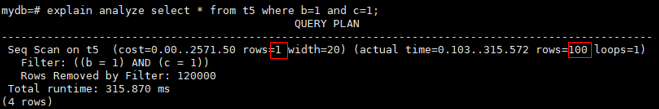--执行explain analyze select * from t5 where b=2 and c=2;并查看计划。真实行数为100000，估算行数为83396，行数估计准确率略低，为0.834。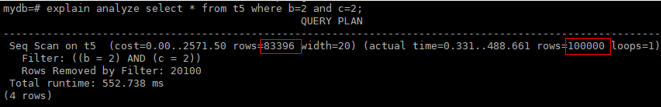--执行explain analyze select * from t5 where b=3 and c=3;10000，估算行数为832，行数估计准确率较低，为0.083。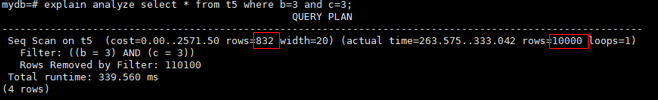--设置ANALYZE的采样方式为百分比采样，采样率为2%set default_statistics_target=-2;--开启GUC参数，生成函数依赖统计信息set enable_functional_dependency=on;alter table t5 add statistics ((a, b, c));analyze t5((a, b, c));--执行explain analyze select * from t5 where b=1 and c=1; 并查看计划。真实行数为100，估算行数为72，所以行数估计准确率为0.72。应用函数依赖统计信息，准确率从0.01提升为0.72，提升72倍。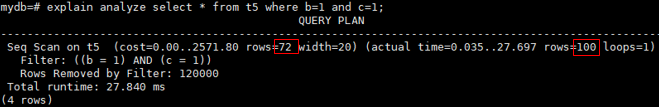--执行explain analyze select * from t5 where b=2 and c=2; 并查看计划。真实行数为100000，估算行数为99708，所以行数估计准确率为0.997。应用函数依赖统计信息，准确率从0.834提升为0.997，提升1.19倍。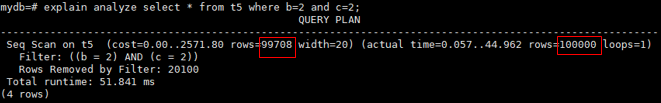--执行explain analyze select * from t5 where b=3 and c=3;10000，估算行数为10066，所以行数估计准确率为0.993。应用函数依赖统计信息，准确率从0.083提升为0.993，提升11.96倍。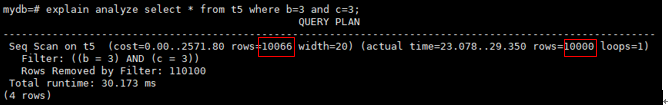帖子
视频
声望
粉丝
相关问题
社区精华内容